Courses
Courses for Kids
Free study material
Free LIVE classes
More

# Normal Form of LineLIVE
Join Vedantu’s FREE Mastercalss

## An Introduction to the Normal Form of Lines

Lines are the basic geometry in mathematics. We have many types of lines as we will learn later in this chapter. As we see every polygon is a set of line segments. And a line is a set of points. Here we will learn how points decide a line. And how we use it in our daily graph calculations.

### What Is Line:

A line is a 1D figure. Which has length but not width. To understand a line we can say that a line is a collection of so many points. And every point is in the opposite direction. Every line has at least two fixed points as a minimum. The line is infinite in both directions. It doesn't have any limits.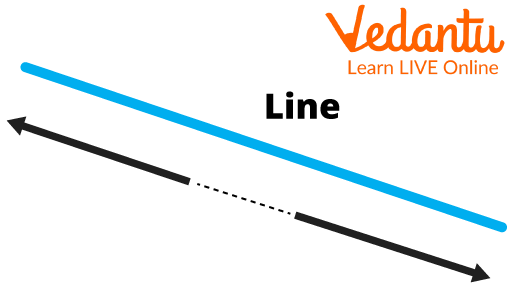Line

Then there is another form of a line which is called a Line segment. It is called a line segment. It is just a part of the line. It has its limits in both directions.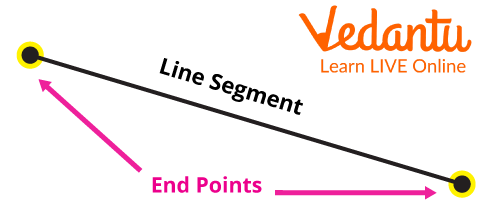Line Segment

The last form of a line is Ray. It is a line that passes through two fixed points in which one point of this line is fixed and the other one is limitless.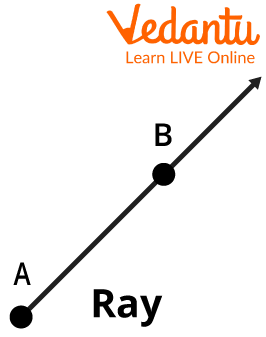Ray

Now, Look at the difference between these all.Ray, Line, Segment

The 1st one is a “Ray”.

2nd is a “Line”.

The 3rd one is the “Line segment”.

### General Equation Of a Straight Line:

It is also known as “The general equation” of a line. We mostly derive it from the help of coordinate geometry.

The equation is:

$y = mx + c$

For example: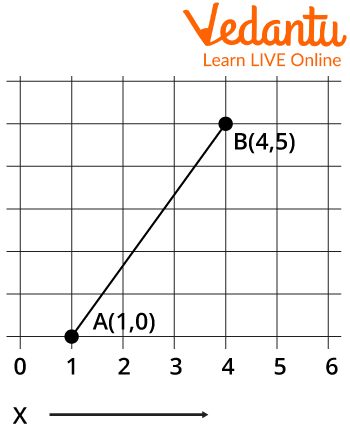Line which has Coordinate A(1,0) and B(4, 5)

We can write its equation as :

Finding slope(m) = $\frac{{5 - 0}}{{4 - 1}} = \frac{5}{3}$

Substituting m into the equation:- y = mx + c

$\Rightarrow y = \frac{5}{3}x + c$

Now put any 1 coordinate value in this equation and find the value of C,

$\Rightarrow 0 = \frac{5}{3} \times 1 + c$

$\Rightarrow - c = \frac{5}{3}$

The equation we get is –

$y = \frac{5}{3}x - \frac{5}{3} \Rightarrow 3y = 5x - 5;$

### The Equation Of Straight Line In Normal Form:

Now let’s get to the normal form of line which is mostly used in geometry.

Here we have an example diagram of normal form derivation.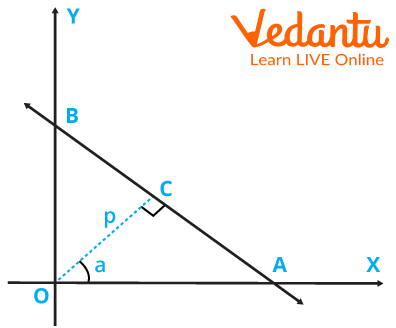A Line Crossing through point A on the X-axis and B on the Y-axis, P is the Perpendicular on AB from the Origin

here, Triangle AOB

P stands for Perpendicular distance from Origin to line AB.

stands for the angle between the perpendicular and X-axis.

Its Equation is:-

$x{\rm{ }}cos{\rm{ \theta}} + y{\rm{}}sin{\rm{ \theta}} = {\rm{ }}p$ Normal Form Formula

### Polar Equation Of Straight Line:

It is called slope-intercept form. As we use a line’s slope and its intercept,

Look we have a general form of a line: ax + by = c

so, to use this equation in the polar form we transform it as $y{\rm{ }} = {\rm{ }}r{\rm{ }}sin\theta$ and $x{\rm{ }} = {\rm{ }}r{\rm{ }}cos\theta$.

The polar equation is –

$\;{\bf{r}}({\bf{a}}{\rm{ }}{\bf{cos}}\theta + {\rm{ }}{\bf{b}}{\rm{ }}{\bf{sin}}\theta ) = {\rm{ }}{\bf{c}}$

We can write polar forms as –

$r\,\sin \theta + mr\,\,\cos \theta + c$

### Solved Examples

Question1: The given figure is an example of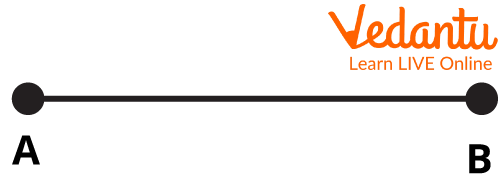• Line Segment

• Perpendicular

• Parallel lines

• Ray

Ans: Line segment

Question2: Identify, which is the straight line –

1.1.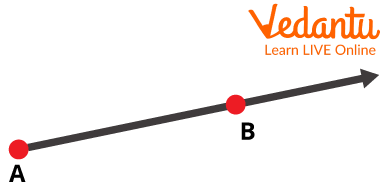1.1.Ans: 3 is the only one extending on both sides to infinity.

### Solved Questions

Question 1: what is the general equation of line?

• My = x + c

• Y = mx + b

• Sin x = 2 + ay

• A = bx + c

[Hint: Y= mx + c]

### Conclusion:

Till now we have learned about lines and the general form of it which is y = mx+c; we now know how to use a line in coordinate. And can derive any line equation from a given coordinate. Let’s have some practice on these concepts now.

Last updated date: 02nd Oct 2023
Total views: 82.8k
Views today: 0.82k

## FAQs on Normal Form of Line

1.Do parallel lines intersect each other?

Parallel lines never intersect each other.

2.Do lines end anywhere?

If you see, Lines expand in each direction endlessly. So theoretically they never end.

3.Why is the distance between 2 parallel lines always equal?

Distance between 2 parallel lines is always equal. Because if they move close or far they will definitely intersect each other at some point.

4.From which line do we take the gradient of a line?

The gradient of a line is generally taken from the x-axis. But we can take it from any axis as per our need.

5.How many dimensions does a line have?

The line has one dimension only, as we required only one coordinate to specify a point on it.